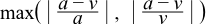#Boost C++ Libraries

...one of the most highly regarded and expertly designed C++ library projects in the world.

This is the documentation for an old version of boost. Click here for the latest Boost documentation.

### Relative Error

Given an actual value a and a found value v the relative error can be calculated from:However the test programs in the library use the symmetrical form:which measures relative difference and happens to be less error prone in use since we don't have to worry which value is the "true" result, and which is the experimental one. It guarantees to return a value at least as large as the relative error.

Special care needs to be taken when one value is zero: we could either take the absolute error in this case (but that's cheating as the absolute error is likely to be very small), or we could assign a value of either 1 or infinity to the relative error in this special case. In the test cases for the special functions in this library, everything below a threshold is regarded as "effectively zero", otherwise the relative error is assigned the value of 1 if only one of the terms is zero. The threshold is currently set at `std::numeric_limits<>::min()`: in other words all denormalised numbers are regarded as a zero.

All the test programs calculate quantized relative error, whereas the graphs in this manual are produced with the actual error. The difference is as follows: in the test programs, the test data is rounded to the target real type under test when the program is compiled, so the error observed will then be a whole number of units in the last place either rounded up from the actual error, or rounded down (possibly to zero). In contrast the true error is obtained by extending the precision of the calculated value, and then comparing to the actual value: in this case the calculated error may be some fraction of units in the last place.

Note that throughout this manual and the test programs the relative error is usually quoted in units of epsilon. However, remember that units in the last place more accurately reflect the number of contaminated digits, and that relative error can "wobble" by a factor of 2 compared to units in the last place. In other words: two implementations of the same function, whose maximum relative errors differ by a factor of 2, can actually be accurate to the same number of binary digits. You have been warned!

##### The Impossibility of Zero Error

For many of the functions in this library, it is assumed that the error is "effectively zero" if the computation can be done with a number of guard digits. However it should be remembered that if the result is a transcendental number then as a point of principle we can never be sure that the result is accurate to more than 1 ulp. This is an example of the table makers dilemma: consider what happens if the first guard digit is a one, and the remaining guard digits are all zero. Do we have a tie or not? Since the only thing we can tell about a transcendental number is that its digits have no particular pattern, we can never tell if we have a tie, no matter how many guard digits we have. Therefore, we can never be completely sure that the result has been rounded in the right direction. Of course, transcendental numbers that just happen to be a tie - for however many guard digits we have - are extremely rare, and get rarer the more guard digits we have, but even so....

Refer to the classic text What Every Computer Scientist Should Know About Floating-Point Arithmetic for more information.